# Pyramid Formula

A polyhedron that has a polygonal base and triangles for sides, is a pyramid. The three main parts of any pyramid’s: apex, face and base. The base of a pyramid may be of any shape. Faces usually take the shape of an isosceles triangle. All the triangles meet at a point on the top of the pyramid that is called “Apex”.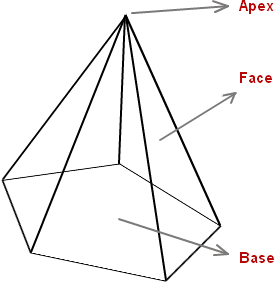The formula for finding the volume and surface area of the pyramid is given as,

$\large Surface\;Area\;of\;a\;Pyramid=Base\;Area+\frac{1}{2}\left(Number\;of\;Base\;Sides \times Slant\;Height \times Base \;Length \right)$
$\large Volume\;of\;a\;Pyramid=\frac{1}{3} \times Base\;Area \times Height$

### Square Pyramid

A Pyramid with a square base, 4 triangular faces and an apex is a square pyramid.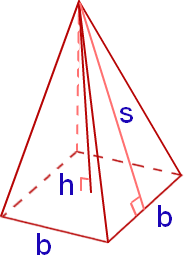The Square Pyramid formulas are,

$\large Base\;Area\;of\;a\;Square\;Pyramid=b^{2}$
$\large Surface\;Area\;of\;a\;Square\;Pyramid=2bs+b^{2}$
$\large Volume\;of\;a\;Square\;Pyramid=\frac{1}{3}b^{2}h$

Where,
b – base length of the square pyramid
s – slant height of the square pyramid
h – height of the square pyramid

### Triangular Pyramid

A triangular pyramid is a type of pyramid with triangular faces and a triangular base.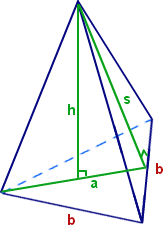The Triangular Pyramid formulas are,

$\large Base\;Area\;of\;a\;Triangular\;Pyramid=\frac{1}{2}\:ab$
$\large Surface\;Area\;of\;a\;Triangular\;Pyramid=\frac{1}{2}\:ab+\frac{3}{2}\:bs$
$\large Volume\;of\;a\;Triangular\;Pyramid=\frac{1}{6}\:abh$

Where,
a – apothem length of the triangular pyramid
b – base length of the triangular pyramid
s – slant height of the triangular pyramid
h – height of the triangular pyramid

### Pentagonal Pyramid

This pyramid has pentagonal base, with 5 sides , triangular faces and an apex.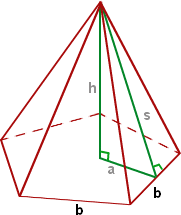The Pentagonal Pyramid Formulas are,

$\large Base\;Area\;of\;a\;Pentagonal\;Pyramid=\frac{5}{2}\:ab$
$\large Surface\;Area\;of\;a\;Pentagonal\;Pyramid=\frac{5}{2}\:ab+\frac{5}{2}\:bs$
$\large Volume\;of\;a\;Pentagonal\;Pyramid=\frac{5}{6}\:abh$

Where,
a – apothem length of the pentagonal pyramid
b – base length of the pentagonal pyramid
s – slant height of the pentagonal pyramid
h – height of the pentagonal pyramid

### Hexagonal Pyramid

This pyramid has a hexagonal base with six sides, six triangular faces and an apex.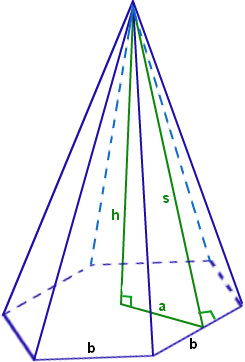The Hexagonal Pyramid Formulas are,

$\large Base\;Area\;of\;a\;Hexagonal\;Pyramid=3ab$
$\large Surface\;Area\;of\;a\;Hexagonal\;Pyramid=3ab+3bs$
$\large Volume\;of\;a\;Hexagonal\;Pyramid=abh$

Where,
a – apothem length of the hexagonal pyramid
b – base length of the hexagonal pyramid
s – slant height of the hexagonal pyramid
h – height of the hexagonal pyramid

#### 1 Comment

1. Asis Kumar De

If we cut the at certain height from the apex point, then what will be the height of the balance cut pyramid. Bottom section is 2070 x 2070, top section is 250 x 250 and volume is 800000000 cubic mm